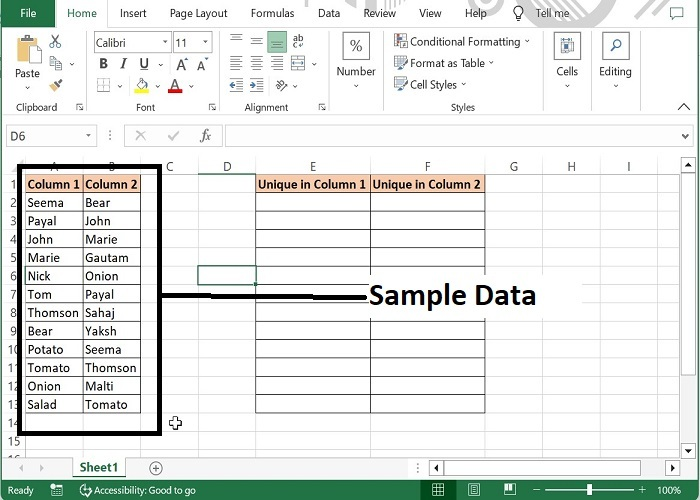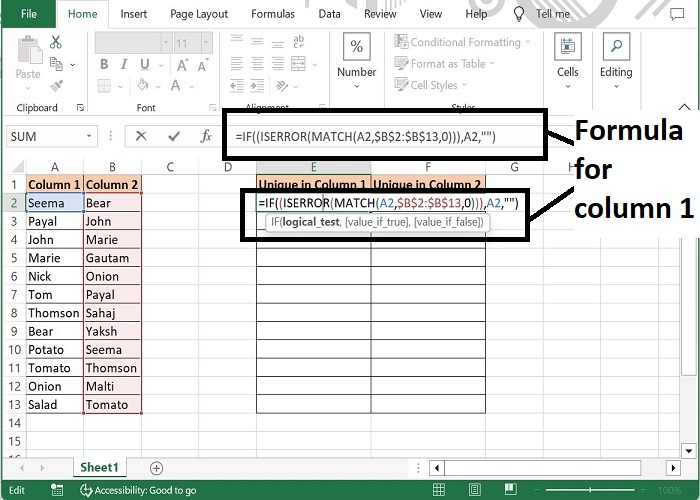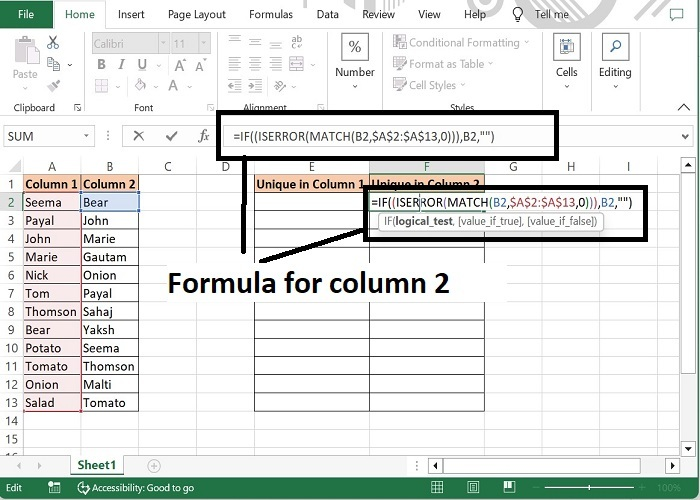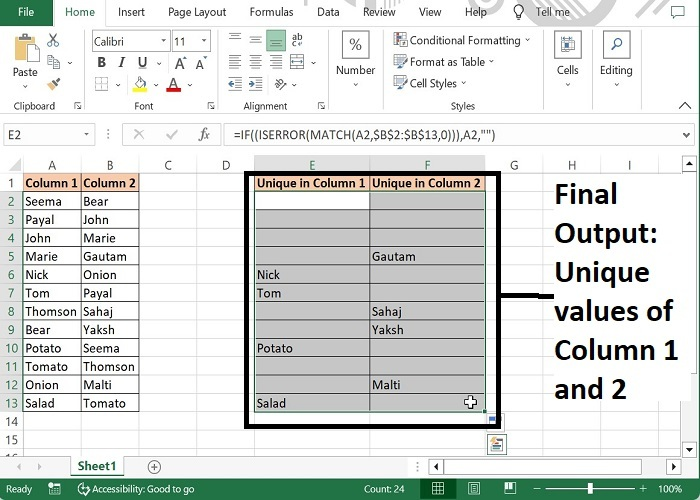# Compare two columns and list differences with formulas.

For instance, we have two columns in a data set having some names. And now we want to identify what names are repeating in either list. For this, we can apply a formula in two other columns, to get the unique values of both the columns. Let’s see how we can achieve this.

## Compare two columns and list differences with formulas

Step 1: We have taken the following sample data for comparison.Step 2: Enter the formula in E2 cell as mentioned below. This formula will return the unique names of column 1, which are not available in column 2.

=IF((ISERROR(MATCH(A2,$B$2:$B$14,0))),A2,"")Step 3: Now, enter the below formula in F2 cell. This formula will return the unique names of column 2, which are not available in column 1.

=IF((ISERROR(MATCH(B2,$A$2:$A$13,0))),B2,"")Step 4: Now, drag the formula till the last row of data and the final output will be as following. The formula will display those names which are unique.### Formula Syntax Description

Argument Description
IF (logical_test, {value_if_true}, {value_if_false})

Logical_test specifies the condition basis which the data needs to be rendered.

Value_if_true specifies the value that shall be returned if the condition satisfies.

Value_if_false specifies the value that shall be returned if the condition does not satisfy.

ISERROR (expression) Expression can be any valid logical formula or value.
MATCH (lookup_value, lookup_array, [match_type])

Lookup value is the value that needs to be matched in look up array. Required

Look up array is the range of cells in which lookup value to be searched. Required

Match type specifies what type of match to be performed. 1 is for larger values, 0 stands for exact values and -1 stands for lesser values.

Note: Here, Unique in Column 1 displays the values which are not available in Column 2 and Unique in Column 2 displays the values which are not available in Column 1.

## Conclusion

Hence using the above formula, value of one column can be returned in another column after comparing with other column. Data of one column can be compared with multiple ranges as well. Keep learning excel.

Updated on: 29-Aug-2023

40 Views